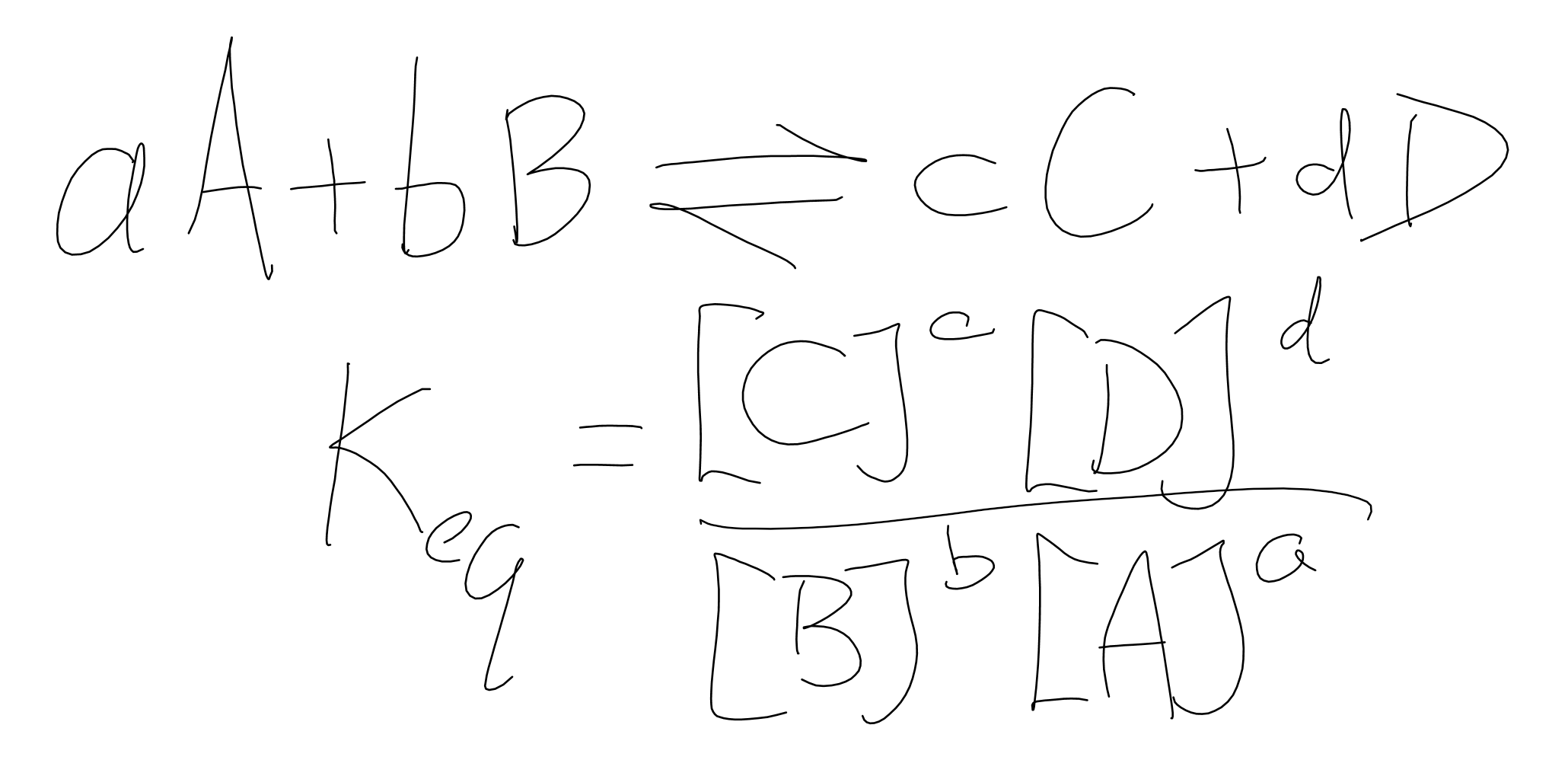Complimentary 1-hour tutoring consultation

MCAT Content / Principles Of Bioenergetics / Bioenergetics Thermodynamics

### Bioenergetics/thermodynamics (BC/GC)

Topic: Principles Of Bioenergetics

A certain ratio of reactants and products for a reaction will always be achieved at equilibrium (assuming temperature is constant). We call this ratio the equilibrium constant, or Keq. It will be equal to the ratio of the concentrations of products to the exponent of their coefficient in the reaction over the concentrations of reactants to the exponent of their coefficient.From there, we can calculate the standard free energy change, ΔG°, for that chemical process at any temperature using the following equation (where R = 8.314 J mol-1 K-1 and T is the temperature in Kelvin).Free energy, called Gibbs free energy (G), is usable energy or energy that is available to do work. Every chemical reaction involves a change in free energy, called delta G (∆G). The change in free energy can be calculated for any system that undergoes a change, such as a chemical reaction. Recall that systems always favour a lower energy state, so when ΔG° is negative, the reaction is spontaneous.

If energy is released during a chemical reaction, then the resulting value from the above equation will be a negative number. In other words, reactions that release energy have a ∆G < 0. A negative ∆G also means that the products of the reaction have less free energy than the reactants because they gave off some free energy during the reaction. Reactions that have a negative ∆G and, consequently, release free energy, are called exothermic reactions. Exergonic means energy is exiting the system. These reactions are also referred to as spontaneous reactions because they can occur without the addition of energy into the system.

If a chemical reaction requires an input of energy rather than releasing energy, then the ∆G for that reaction will be a positive value. In this case, the products have more free energy than the reactants. Thus, the products of these reactions can be thought of as energy-storing molecules. These chemical reactions are called endothermic reactions; they are nonspontaneous. An endergonic reaction will not take place on its own without the addition of free energy.

The significance of the sign of a change in Gibbs free energy parallels the relationship of Keq when examining spontaneous and nonspontaneous reactions

If K, the reaction is nonspontaneous in the direction written.

If = K, the reaction is in a state of equilibrium.

If K, the reaction is spontaneous in the direction written.

Lastly, Le Châtelier’s Principle describes factors that will affect the equilibrium of a system. It basically describes how the system will respond to stress. Here is a summary of factors you should know:

• Increasing the concentration of reactants will drive the reaction to the right towards the products, while increasing the concentration of products will drive the reaction to the left towards the reactants.
• An increase in pressure or decrease in volume will result in a shift to the side with fewer moles of gas. Conversely, an decrease in pressure or increase in volume will result in a shift in to the side with more moles of gas.
• An inert gas like argon will have no effect on equilibrium.

Practice Questions

Control of glucose levels

Basic concepts in bioenergetics: phosphoryl group transfers and ATP hydrolysis

The thermodynamics of ATP hydrolysis in living cells

Heat engines and work

MCAT Official Prep (AAMC)

Official Guide C/P Section Passage 1 Question 4

Practice Exam 1 B/B Section Passage 3 Question 17

Key Points

• The equilibrium constant (Keq) is equal to the ratio of products over reactants. It can then be used to calculate the standard free energy change.

• When ΔG° is negative, the reaction is spontaneous.

• Endothermic reactions have heat as a reactant while exothermic reactions have heat as a product.

• Le Châtelier’s Principle describes how factors affect a system’s equilibrium.

• Endothermic reactions require an input of energy; the ∆G for that reaction will be a positive value.

• Exothermic reactions release free energy; the ∆G for that reaction will be a negative value.

Key Terms

free energy: change of energy due to non-expansion work from a system at a constant temperature and pressure

Le Châtelier’s Principle: describes factors that will affect the equilibrium of a system

equilibrium constant (Keq): the ratio of the mathematical product of the concentrations of the products of a reaction to the mathematical product of the concentrations of the reactants of the reaction.

exothermic: a chemical reaction where the change in the Gibbs free energy is negative, indicating a spontaneous reaction

endothermic: a chemical reaction in which the standard change in free energy is positive, and energy is absorbed

Gibbs free energy: the difference between the enthalpy of a system and the product of its entropy and absolute temperature

Billing Information